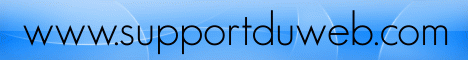# stats_cdf_beta

(PECL stats:1.0.0-1.0.2)

stats_cdf_beta — Fonction CDF pour Distribution BETA. Calcule n'importe quel paramètre de distribution beta des valeurs données pour les autres.

### Description

float stats_cdf_beta ( float \$par1 , float \$par2 , float \$par3 , int \$which )

```                              Method

Cumulative distribution function  (P)  is calculated directly by
code associated with the following reference.

DiDinato, A. R. and Morris,  A.   H.  Algorithm 708: Significant
Digit Computation of the Incomplete  Beta  Function Ratios.  ACM
Trans. Math.  Softw. 18 (1993), 360-373.

Computation of other parameters involve a seach for a value that
produces  the desired  value  of P.   The search relies  on  the
monotinicity of P with the other parameter.

Note

The beta density is proportional to
t^(A-1) * (1-t)^(B-1)

Arguments

P -- The integral from 0 to X of the chi-square
distribution.
Input range: [0, 1].

Q -- 1-P.
Input range: [0, 1].
P + Q = 1.0.

X -- Upper limit of integration of beta density.
Input range: [0,1].
Search range: [0,1]

Y -- 1-X.
Input range: [0,1].
Search range: [0,1]
X + Y = 1.0.

A -- The first parameter of the beta density.
Input range: (0, +infinity).
Search range: [1D-100,1D100]

B -- The second parameter of the beta density.
Input range: (0, +infinity).
Search range: [1D-100,1D100]

STATUS  -- 0 if calculation completed correctly
-I if input parameter number I is out of range
1 if answer appears to be lower than lowest
search bound
2 if answer appears to be higher than greatest
search bound
3 if P + Q .ne. 1
4 if X + Y .ne. 1

BOUND  -- Undefined if STATUS is 0

Bound exceeded by parameter number I if STATUS
is negative.

Lower search bound if STATUS is 1.

Upper search bound if STATUS is 2.
```

### Liste de paramètres

par1

par2

par3

which

```      Integer indicating which of the next four argument
values is to be calculated from the others.
Legal range: 1..4
iwhich = 1 : Calculate P and Q from X,Y,A and B
iwhich = 2 : Calculate X and Y from P,Q,A and B
iwhich = 3 : Calculate A from P,Q,X,Y and B
iwhich = 4 : Calculate B from P,Q,X,Y and A

```

### Valeurs de retour

```     STATUS  -- 0 if calculation completed correctly
-I if input parameter number I is out of range
1 if answer appears to be lower than lowest
search bound
2 if answer appears to be higher than greatest
search bound
3 if P + Q .ne. 1
4 if X + Y .ne. 1
```

Ceci n'est pas la documentation originale du langage de programmation php, pour y accéder visiter le site www.php.netPage générée en 0.001491 secondes.